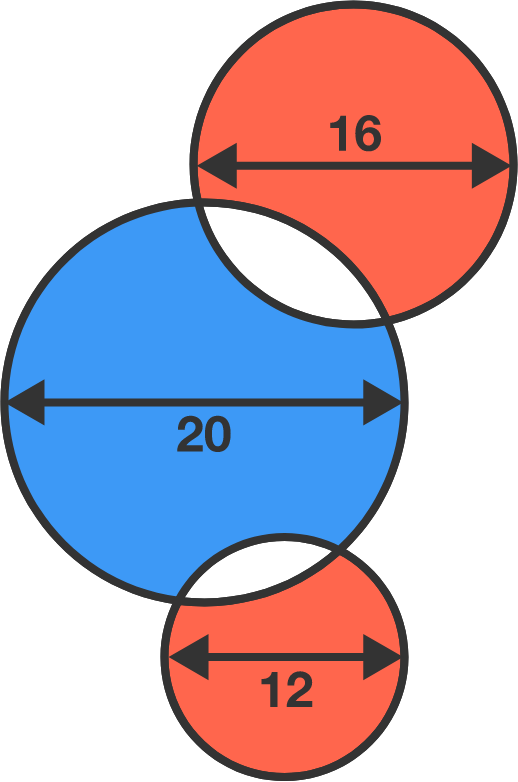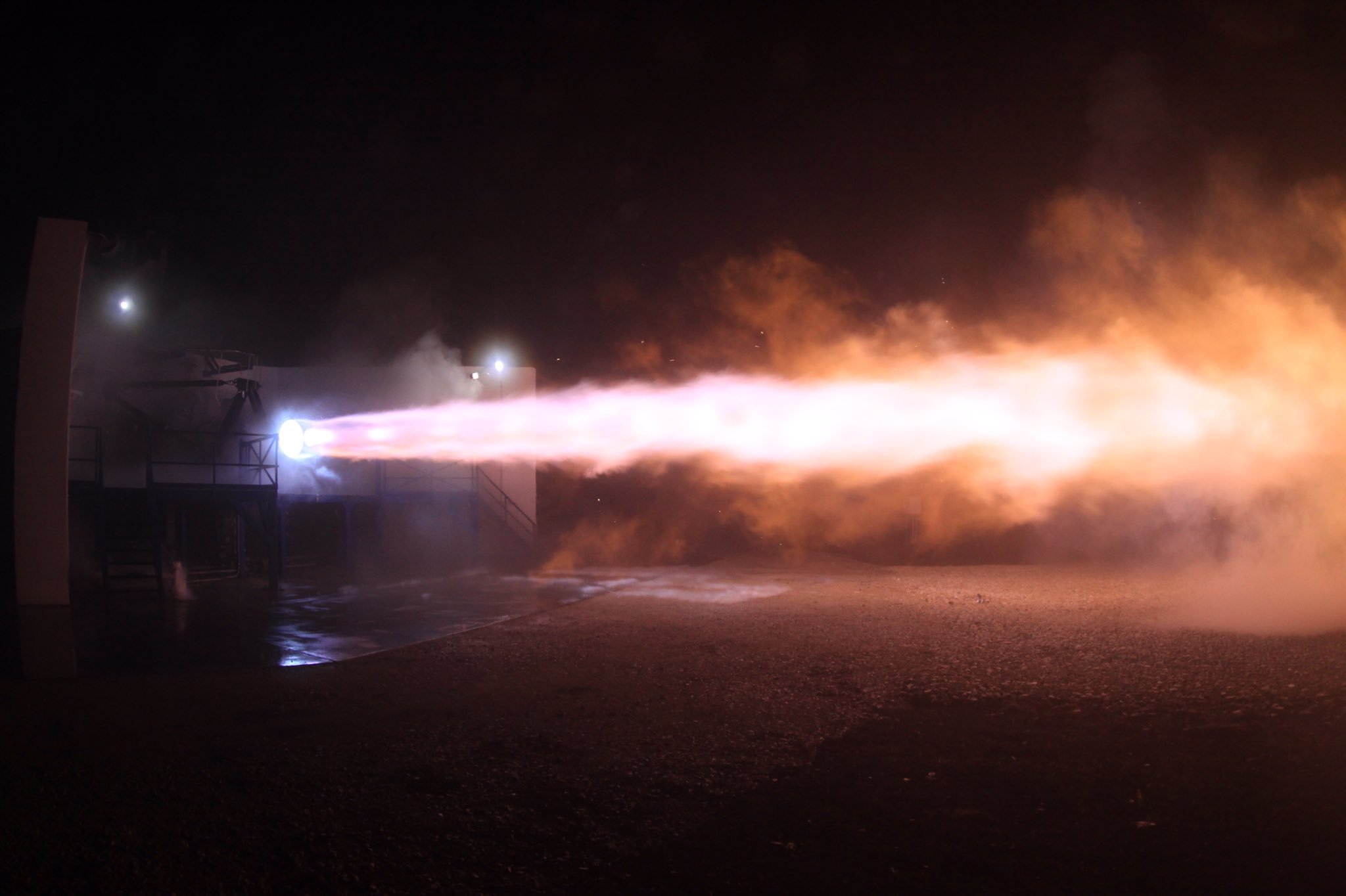# Problems of the Week

Contribute a problem

# 2018-04-30 Intermediate$B$ is the area of the blue region, and $R$ is the area of the red region.

Which statement is correct?

The distance between any two adjacent cars at a red light is 2 meters.

As soon as the light turns green, the first car starts accelerating at $\SI{4}{m/s^2}.$ As soon as the first car reaches a speed of $\SI{6}{m/s},$ the second car starts accelerating at the same rate. In exactly the same fashion, each successive car starts accelerating when the car in front of it reaches $\SI{6}{m/s},$ and accelerates at $\SI{4}{m/s^2}$ until it reaches the speed $\SI{20}{m/s}.$

When all cars have reached the same speed $\SI{20}{m/s},$ how far apart are two adjacent cars (in meters)?For two random positive real numbers $x$ and $y$ chosen uniformly and independently from the interval $(1,1000)$, determine the probability that $\lfloor x+y \rfloor = \lfloor x \rfloor + \lfloor y \rfloor.$

Notation: $\lfloor \cdot \rfloor$ denotes the floor function.

The equation of motion for rockets is $m_\textrm{r}\,\dot{v}_\textrm{r}= u_\textrm{e}\, \dot{m}_\textrm{r},$ where $u_\textrm{e}$ is the speed of the exhaust shooting out the back. The final velocity of the rocket is a few multiples of the exhaust speed, so it's quite important, but in physics textbooks, $u_\textrm{e}$ is usually treated like a freely adjustable parameter whose origin and precise value is a detail left for engineers to worry about. With a little bit of approximation, we can do better than that.

Estimate $u_\textrm{e}$ for the combustion of methane $(\ce{CH4}),$ the propellant used by SpaceX for its Raptor rocket engine program.Details and Assumptions:

• The specific enthalpy of combustion for methane is $\Delta_c H \approx \SI[per-mode=symbol]{890}{\kilo\joule\per\mole}.$
• Assume that all the energy released in combustion goes into the kinetic energy of the gas.
• Beyond its performance, methane is nice because it could potentially be produced on other planets via the Sabatier reaction $\ce{CO2 + 4H2 -> CH4 + 2H2O}.$

$\big(x - 1\big)\big(x^2 - 2\big)\big(x^3 - 3\big)\cdots \big(x^{20} - 20\big)$

What is the coefficient of $x^{203}$ in the expansion of this expression?

×